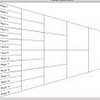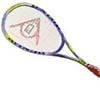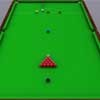You may also likeKnock-out

Before a knockout tournament with 2^n players I pick two players. What is the probability that they have to play against each other at some point in the tournament?Squash

If the score is 8-8 do I have more chance of winning if the winner is the first to reach 9 points or the first to reach 10 points?Snooker

A player has probability 0.4 of winning a single game. What is his probability of winning a 'best of 15 games' tournament?

Dicey Decisions

Why do this problem?

This problem explores the familiar context of probability with dice, in an unfamiliar way.

Possible approach

This problem is set in the same context as the Stage 3 problem Troublesome Dice

Make sure students understand the standard way of labelling the faces of a six-sided die. They will need to calculate all the possible edge and corner totals in order to calculate the relative frequencies. There are different areas for exploration suggested in the problem - one possibility is to assign particular values to the probability of landing face, edge, and corner up. Values of $\frac{1}{2}$,$\frac{1}{3}$ and $\frac{1}{6}$ are suggested - students could compute the overall probabilities for each total. Alternatively, the general case with probabilities $p$, $q$ and $r = 1 - (p + q)$ could be explored - this would work well in small groups with students sharing out the work.

Once students have computed the probability of each total (either for specific values or in terms of $p$ and $q$), challenge them to find fair ways of allocating the numbers for different sizes of family. They could work in small groups and present their solutions to the rest of the class.

Key questions

I can get a 6 as a face-up, edge-up or corner-up score. Which scores would you want to be allocated to balance this out?
A 6-sided die can be labelled in a "left-handed" or "right-handed" manner - do the two dice give different answers?
The probabilities are distributed symmetrically for the face, edge and corner sums. Is this surprising? Can you explain why?

Possible extension

Consideration of the probabilities for dodecahedral or icosahedral dice provide a challenging extension.

Possible support

Spend some time working on Troublesome Dice to make sure students are happy with the relative probabilities of the edge-up and corner-up scores.